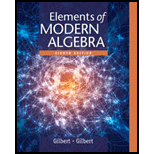# Whether the statement, “A p − group can be finite or infinite”, is true or false.### Elements Of Modern Algebra

8th Edition
Gilbert + 2 others
Publisher: Cengage Learning,
ISBN: 9781285463230### Elements Of Modern Algebra

8th Edition
Gilbert + 2 others
Publisher: Cengage Learning,
ISBN: 9781285463230

#### Solutions

Chapter 4.8, Problem 1TFE
To determine

## Whether the statement, “A p− group can be finite or infinite”, is true or false.

Expert Solution

Solution:

The statement, “A p group can be finite or infinite”, is True.

### Explanation of Solution

Formula used:

If p is prime, then group G is called a p group if and only if each of its elements has an order that is a power of p.

Explanation:

Consider the given statement, “A p group can be finite or infinite”.

Consider the additive abelian group 22={(0,0),(0,1),(1,0),(1,1)} of order 4.

Every element in the group 22 has order power of 2.

By using the definition of p group, 22 is 2 group.

Therefore, 22 is an example of finite p group.

If a finite group is p group, then there are finite terms of power p.

Now, consider additive abelian group 2222......... of infinite order.

Every element in the group 2222......... has order power of 2.

By using the definition of p group, 2222......... is 2 group.

Therefore, 2222......... is an example of infinite p group.

If an infinite group is a p group, then there are finite or infinite terms of power p.

Therefore, the statement, “A p group can be finite or infinite” is true.

### Want to see more full solutions like this?

Subscribe now to access step-by-step solutions to millions of textbook problems written by subject matter experts!

### Want to see more full solutions like this?

Subscribe now to access step-by-step solutions to millions of textbook problems written by subject matter experts!